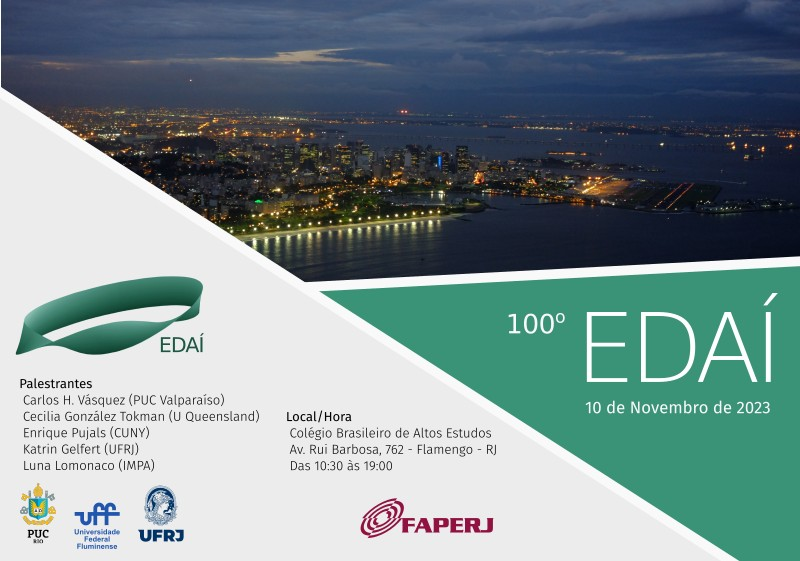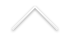100º EDAÍ, 10/11 no CBAE em Flamengo

Local: Colégio Brasileiro de Altos Estudos, Av. Rui Barbosa, 762 - Flamengo

Data e hora: 10 de novembro de 2023, das 10:30 às 18:30

10h30 – 11h30: Katrin Gelfert (UFRJ)

Ergodic recycling of heterodimensional cycles

We define the concept of heterodimensional cycles between hyperbolic ergodic measures of different indices. We study what impact the existence of such a cycle has on the topological properties of the space of invariant measures and present some examples. This is joint work with L. Díaz and Ch. Bonatti.

11h45 - 12h45: Carlos H. Vásquez (PUC Valparaíso)

Measures of maximal entropy for partially hyperbolic diffeomorphisms.

A central problem in dynamics is being able to determine the existence of measures that capture relevant dynamic information. One of these measures is the one that maximizes entropy. In this talk, we will explore the problem of establishing the existence and uniqueness of this measure in partially hyperbolic systems.

14h30 - 15h30: Luna Lomonaco (IMPA)

The Mandelbrot set and its Satellite copies

For a polynomial on the Riemann sphere, infinity is a (super) attracting fixed point, and the filled Julia set is the set of points with bounded orbit. Consider the quadratic family P_c(z)=z^2+c. The Mandelbrot set M is the set of parameters c such that the filled Julia set of P_c is connected.

Computer experiments quickly reveal the existence of small homeomorphic copies of M inside itself; the existence of such copies was proved by Douady and Hubbard. Each little copy is either primitive (with a cusp on the boundary of its main cardioid region) or a satellite (without a cusp). Lyubich proved that the primitive copies of M satisfy a stronger regularity condition: they are quasiconformally homeomorphic to M. The satellite copies are not quasiconformally homeomorphic to M (as we cannot straighten a cusp quasiconformally), but are they mutually quasiconformally homeomorphic? In joint work with C. Petersen we prove that the answer is negative in general, but positive in the case the satellite copies have rotation number with same denominator (this last part is work in progress).

15h40 - 16h40: Cecilia González Tokman (University of Queensland - UQ)

Lyapunov–Oseledets spectrum for transfer operator cocycles under perturbations

In recent years, the study of transfer operators has been combined with multiplicative ergodic theory to shed light on ergodic-theoretic properties of random dynamical systems. The so-called Lyapunov– Oseledets spectrum associated to the transfer operator cocycle contains fundamental information about invariant measures, exponential decay rates and coherent structures which characterize dominant global transport features of the system. While the scope of this framework is broad, it is challenging to identify and approximate this spectrum. In this talk, we present examples of maps where the Lyapunov– Oseledets spectrum can be understood and analyzed under perturbations. This talk is based on joint work with Anthony Quas.

17h10 – 18h10: Enrique Pujals (City University of New York - CUNY)

From zero to positive entropy

We will discuss the mathematical processes by which a system evolves from one whose recurrent set is finite towards another one exhibiting chaotic behavior as parameters governing the behavior of the system are varied. In that direction, we will present a tentative global framework toward describing a large class of two-dimensional dynamics (that includes the Henon family), inspired partially by the developments in the one-dimensional theory of interval maps. More precisely, we present a class of intermediate smooth dynamics between one and higher dimensions where it is possible to describe the transition from zero to positive entropy.